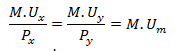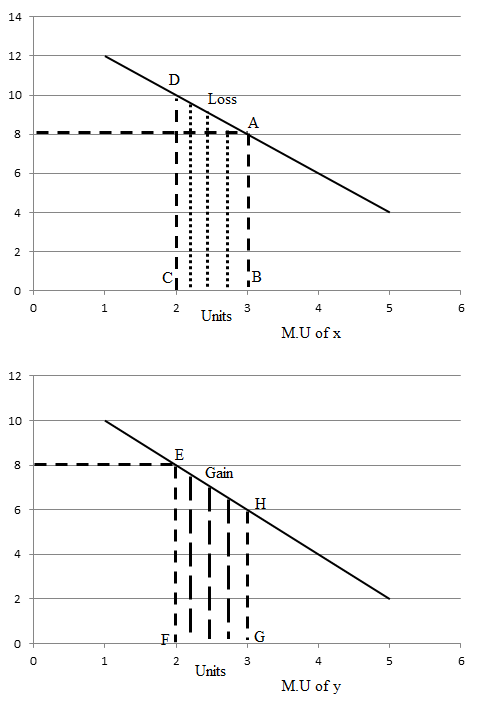## TyroCity

Economics 12 Notes for Economics Notes

Posted on • Updated on

# The law of maximum satisfaction/ The law of equi-marginal utility/ The law of substitution

The law of maximum satisfaction/ The law of equi-marginal utility/ The law of substitution.

This law is developed by H.H Gossen so it is also called the second law of Gossen. We know human wants are unlimited but the resources to fulfill the wants are limited. A rational consumer always tries to maximize his satisfaction by spending his limited money income. Consumer can maximize his satisfaction if he is able to equalize the marginal utility derived from the consumption of different units of several commodities by spending his all limited money income so that this law is known as law of maximum satisfaction or law of equi-marginal utility.

This law is also known as law of substitution because consumer can maximize his/her satisfaction when he/she substitutes the commodities having high marginal utility instead of commodities having the low marginal utility.

Mathematically it is expressed as:This law is based on the following assumptions:

1. Consumers should be rational
2. Price of commodity remains constant
3. Income of consumers remains constant
4. Utility can be measured in numbers
5. Marginal utility of money remains constant

We can describe this theory by the help of given table and figure:

Suppose income of consumer is Rs 50. There are two commodities i.e. x and y for consumption. Price of per unit commodity is Rs 10.

 Units M.U of x M.U of y 1 12 10 2 10 5 3 8 6 4 6 4 5 4 2 Total = 40 Total = 30

On the above table by using Rs 50 consumer can consume all five units of x or five units of y or combination of both. When he spends all his income on x he gets 40 as total utility and if he spends all his income on y he gets 30 as total utility. But if he spends his money income on the combination of both i.e. 3 units of x and 2 units of y he gets 48 as total utility which is the maximum satisfaction than any other combination. In this situation utility derived from last units is equal i.e. 8.

The same concept can be explained by given figure:On the above figure M.U and units of commodity is measured along the y and x-axes. When consumer consumes 3rd coordinate of x he gets AB=M.U and when he consumes second unit of y he gets M.U equal to EF where AB=EF. In these situation consumers gets maximum satisfaction by spending his limited money.

Suppose, consumer consumes FG amount of y commodity at that time he must reduce the consumption of x FG=BC amount. At that time he gets utility area equal to EFGH from y, and he losses the utility area equal to ABCD from x. The area ABCD is greater than the area EFGH. It means consumer losses more and gains less. So, maximum satisfaction is possible only when there is equality of marginal utility from different units of same commodity.